### SupernovaHover over the thumbnail for a full-size version.

Author Evil___ author:evil___ autogenerated featured n-art rated 2006-04-29 4 by 20 people. \$Supernova#Evil___#none#00000000000000000000000000000000000000000000000000000000000000000000000000000000000000000000000000000000000000000000000000000000000000000000000000000000000000000000000000000000000000000000000000000000000000000000000000000000000000000000000000000000000000000000000000000000000000000000000000000000000000000000000000000000000000000000000000000000000000000000000000000000000000000000000000000000000000000000000000000000000000000000000000000000000000000000000000000000000000000000000000000000000000000000000000000000000000000000000000000000000000000000000000000000000000000000000000000000000000000000000000000000000000000000000000000000000000000000000000000000000000000000000000000000000000000000000000000000000000000|0^780,588!12^24,276!12^24,336!12^30,210!12^30,288!12^30,300!12^30,378!12^36,108!12^36,402!12^36,420!12^36,480!12^42,228!12^42,246!12^42,378!12^48,180!12^48,288!12^48,300!12^48,330!12^48,342!12^48,360!12^48,432!12^48,450!12^54,216!12^60,252!12^60,276!12^60,294!12^60,300!12^60,312!12^60,318!12^60,336!12^60,348!12^60,360!12^60,390!12^72,192!12^72,210!12^72,222!12^72,240!12^72,288!12^72,300!12^72,336!12^72,348!12^72,366!12^72,396!12^78,108!12^78,168!12^78,198!12^78,228!12^78,294!12^78,312!12^78,348!12^78,378!12^78,384!12^78,438!12^84,120!12^84,180!12^84,288!12^84,294!12^84,312!12^84,336!12^84,342!12^84,450!12^84,510!12^90,192!12^90,210!12^90,246!12^90,258!12^90,282!12^90,300!12^90,366!12^90,384!12^96,66!12^96,132!12^96,174!12^96,198!12^96,210!12^96,222!12^96,246!12^96,264!12^96,276!12^96,306!12^96,336!12^96,348!12^96,366!12^96,372!12^96,396!12^96,402!12^102,120!12^102,138!12^102,252!12^102,264!12^102,288!12^102,294!12^102,300!12^102,306!12^102,330!12^102,342!12^102,456!12^102,474!12^108,156!12^108,252!12^108,288!12^108,300!12^108,336!12^108,432!12^114,108!12^114,138!12^114,174!12^114,180!12^114,204!12^114,210!12^114,216!12^114,234!12^114,264!12^114,336!12^114,366!12^114,384!12^114,390!12^114,396!12^114,426!12^114,432!12^114,444!12^114,462!12^114,468!12^114,498!12^120,168!12^120,240!12^120,252!12^120,258!12^120,288!12^120,294!12^120,336!12^120,342!12^120,372!12^120,384!12^120,456!12^120,462!12^126,90!12^126,102!12^126,126!12^126,180!12^126,192!12^126,252!12^126,288!12^126,294!12^126,306!12^126,312!12^126,318!12^126,324!12^126,342!12^126,408!12^126,456!12^126,528!12^126,540!12^132,120!12^132,186!12^132,198!12^132,222!12^132,228!12^132,246!12^132,258!12^132,264!12^132,270!12^132,282!12^132,294!12^132,318!12^132,324!12^132,342!12^132,348!12^132,354!12^132,360!12^132,366!12^132,384!12^132,390!12^132,396!12^132,420!12^138,174!12^138,180!12^138,186!12^138,192!12^138,198!12^138,264!12^138,282!12^138,288!12^138,312!12^138,324!12^138,402!12^138,408!12^138,414!12^138,420!12^138,438!12^144,120!12^144,126!12^144,156!12^144,198!12^144,204!12^144,210!12^144,222!12^144,234!12^144,264!12^144,276!12^144,288!12^144,294!12^144,300!12^144,312!12^144,318!12^144,354!12^144,366!12^144,378!12^144,390!12^144,432!12^144,462!12^150,180!12^150,204!12^150,222!12^150,234!12^150,252!12^150,258!12^150,288!12^150,300!12^150,318!12^150,348!12^150,354!12^150,366!12^150,378!12^150,390!12^150,420!12^150,450!12^156,90!12^156,198!12^156,222!12^156,228!12^156,246!12^156,264!12^156,282!12^156,288!12^156,306!12^156,318!12^156,324!12^156,336!12^156,342!12^156,360!12^156,366!12^156,378!12^156,384!12^156,402!12^156,474!12^156,534!12^162,90!12^162,120!12^162,126!12^162,132!12^162,168!12^162,204!12^162,216!12^162,240!12^162,246!12^162,252!12^162,258!12^162,270!12^162,276!12^162,294!12^162,312!12^162,318!12^162,324!12^162,342!12^162,360!12^162,366!12^162,378!12^162,384!12^162,390!12^162,396!12^162,414!12^162,420!12^162,432!12^162,468!12^162,504!12^162,516!12^162,546!12^168,108!12^168,120!12^168,150!12^168,156!12^168,186!12^168,192!12^168,198!12^168,204!12^168,222!12^168,234!12^168,246!12^168,258!12^168,264!12^168,276!12^168,282!12^168,288!12^168,294!12^168,306!12^168,312!12^168,324!12^168,330!12^168,336!12^168,348!12^168,354!12^168,366!12^168,372!12^168,384!12^168,390!12^168,396!12^168,402!12^168,420!12^168,426!12^168,432!12^168,438!12^168,462!12^168,468!12^168,474!12^168,492!12^168,516!12^174,120!12^174,138!12^174,144!12^174,150!12^174,192!12^174,198!12^174,204!12^174,210!12^174,228!12^174,240!12^174,246!12^174,252!12^174,258!12^174,294!12^174,300!12^174,336!12^174,348!12^174,354!12^174,366!12^174,372!12^174,384!12^174,396!12^174,402!12^174,408!12^174,450!12^174,456!12^174,474!12^180,60!12^180,66!12^180,96!12^180,108!12^180,120!12^180,126!12^180,132!12^180,138!12^180,156!12^180,162!12^180,168!12^180,186!12^180,192!12^180,198!12^180,204!12^180,210!12^180,228!12^180,234!12^180,240!12^180,246!12^180,258!12^180,276!12^180,282!12^180,294!12^180,306!12^180,330!12^180,336!12^180,342!12^180,348!12^180,354!12^180,360!12^180,378!12^180,384!12^180,390!12^180,396!12^180,402!12^180,420!12^180,426!12^180,432!12^180,444!12^180,456!12^180,462!12^180,468!12^180,480!12^180,486!12^180,492!12^180,522!12^186,132!12^186,138!12^186,144!12^186,150!12^186,156!12^186,174!12^186,180!12^186,198!12^186,210!12^186,216!12^186,222!12^186,228!12^186,234!12^186,240!12^186,246!12^186,252!12^186,258!12^186,276!12^186,282!12^186,294!12^186,300!12^186,306!12^186,318!12^186,342!12^186,348!12^186,354!12^186,360!12^186,372!12^186,378!12^186,384!12^186,390!12^186,402!12^186,420!12^186,444!12^186,450!12^186,456!12^186,462!12^186,522!12^186,546!12^192,66!12^192,96!12^192,102!12^192,126!12^192,138!12^192,150!12^192,186!12^192,204!12^192,216!12^192,222!12^192,228!12^192,246!12^192,258!12^192,264!12^192,270!12^192,282!12^192,294!12^192,300!12^192,306!12^192,312!12^192,318!12^192,324!12^192,330!12^192,342!12^192,354!12^192,360!12^192,366!12^192,378!12^192,396!12^192,402!12^192,408!12^192,414!12^192,420!12^192,438!12^192,474!12^192,486!12^192,498!12^192,522!12^192,528!12^192,558!12^198,150!12^198,156!12^198,162!12^198,168!12^198,174!12^198,180!12^198,186!12^198,192!12^198,204!12^198,210!12^198,240!12^198,246!12^198,252!12^198,264!12^198,270!12^198,276!12^198,282!12^198,288!12^198,294!12^198,300!12^198,306!12^198,312!12^198,318!12^198,324!12^198,330!12^198,336!12^198,342!12^198,348!12^198,354!12^198,366!12^198,372!12^198,378!12^198,384!12^198,390!12^198,396!12^198,426!12^198,432!12^198,444!12^198,450!12^198,456!12^198,462!12^198,468!12^198,474!12^198,480!12^198,486!12^204,36!12^204,72!12^204,102!12^204,108!12^204,120!12^204,132!12^204,138!12^204,144!12^204,156!12^204,162!12^204,186!12^204,192!12^204,198!12^204,222!12^204,234!12^204,240!12^204,246!12^204,252!12^204,258!12^204,264!12^204,270!12^204,282!12^204,288!12^204,294!12^204,300!12^204,306!12^204,318!12^204,324!12^204,330!12^204,336!12^204,342!12^204,348!12^204,354!12^204,360!12^204,366!12^204,372!12^204,378!12^204,384!12^204,390!12^204,396!12^204,402!12^204,426!12^204,432!12^204,438!12^204,462!12^204,468!12^204,480!12^204,486!12^204,492!12^204,504!12^204,516!12^210,30!12^210,36!12^210,54!12^210,84!12^210,156!12^210,174!12^210,180!12^210,192!12^210,210!12^210,216!12^210,222!12^210,228!12^210,234!12^210,240!12^210,246!12^210,252!12^210,258!12^210,264!12^210,270!12^210,282!12^210,288!12^210,294!12^210,300!12^210,306!12^210,312!12^210,318!12^210,330!12^210,336!12^210,342!12^210,348!12^210,354!12^210,360!12^210,366!12^210,372!12^210,378!12^210,384!12^210,390!12^210,420!12^210,426!12^210,444!12^210,546!12^210,564!12^210,570!12^216,84!12^216,96!12^216,102!12^216,114!12^216,120!12^216,126!12^216,138!12^216,156!12^216,168!12^216,174!12^216,180!12^216,186!12^216,192!12^216,198!12^216,204!12^216,216!12^216,222!12^216,228!12^216,240!12^216,246!12^216,252!12^216,258!12^216,264!12^216,270!12^216,276!12^216,282!12^216,288!12^216,300!12^216,306!12^216,312!12^216,318!12^216,324!12^216,330!12^216,336!12^216,342!12^216,348!12^216,354!12^216,360!12^216,366!12^216,372!12^216,384!12^216,390!12^216,396!12^216,408!12^216,414!12^216,426!12^216,432!12^216,450!12^216,462!12^216,474!12^216,486!12^216,492!12^216,504!12^222,42!12^222,78!12^222,102!12^222,114!12^222,120!12^222,126!12^222,132!12^222,144!12^222,156!12^222,162!12^222,168!12^222,174!12^222,180!12^222,186!12^222,192!12^222,210!12^222,216!12^222,222!12^222,228!12^222,234!12^222,240!12^222,246!12^222,252!12^222,258!12^222,264!12^222,270!12^222,276!12^222,282!12^222,288!12^222,294!12^222,300!12^222,306!12^222,312!12^222,318!12^222,324!12^222,330!12^222,336!12^222,342!12^222,348!12^222,354!12^222,360!12^222,366!12^222,372!12^222,378!12^222,384!12^222,390!12^222,402!12^222,408!12^222,414!12^222,420!12^222,426!12^222,432!12^222,438!12^222,444!12^222,456!12^222,468!12^222,474!12^222,480!12^222,486!12^222,516!12^228,36!12^228,78!12^228,90!12^228,138!12^228,144!12^228,150!12^228,168!12^228,180!12^228,186!12^228,192!12^228,198!12^228,204!12^228,210!12^228,216!12^228,222!12^228,228!12^228,234!12^228,240!12^228,246!12^228,252!12^228,258!12^228,264!12^228,270!12^228,276!12^228,288!12^228,294!12^228,300!12^228,306!12^228,312!12^228,318!12^228,324!12^228,330!12^228,342!12^228,348!12^228,354!12^228,360!12^228,366!12^228,372!12^228,378!12^228,384!12^228,390!12^228,396!12^228,402!12^228,408!12^228,414!12^228,420!12^228,426!12^228,432!12^228,438!12^228,444!12^228,456!12^228,468!12^228,474!12^228,480!12^228,486!12^228,528!12^228,534!12^228,546!12^228,552!12^234,36!12^234,102!12^234,132!12^234,138!12^234,156!12^234,162!12^234,168!12^234,174!12^234,180!12^234,186!12^234,192!12^234,198!12^234,210!12^234,216!12^234,222!12^234,228!12^234,234!12^234,240!12^234,246!12^234,252!12^234,258!12^234,264!12^234,270!12^234,276!12^234,282!12^234,288!12^234,294!12^234,300!12^234,306!12^234,312!12^234,318!12^234,324!12^234,330!12^234,336!12^234,342!12^234,348!12^234,354!12^234,360!12^234,366!12^234,372!12^234,378!12^234,384!12^234,390!12^234,396!12^234,402!12^234,408!12^234,414!12^234,420!12^234,426!12^234,438!12^234,444!12^234,450!12^234,456!12^234,462!12^234,468!12^234,474!12^234,480!12^234,498!12^234,504!12^240,30!12^240,66!12^240,84!12^240,102!12^240,108!12^240,120!12^240,126!12^240,132!12^240,150!12^240,156!12^240,162!12^240,168!12^240,174!12^240,180!12^240,198!12^240,204!12^240,210!12^240,222!12^240,240!12^240,246!12^240,252!12^240,258!12^240,270!12^240,276!12^240,282!12^240,288!12^240,294!12^240,300!12^240,306!12^240,312!12^240,318!12^240,324!12^240,330!12^240,336!12^240,342!12^240,348!12^240,354!12^240,366!12^240,372!12^240,378!12^240,384!12^240,414!12^240,420!12^240,426!12^240,450!12^240,456!12^240,462!12^240,468!12^240,474!12^240,486!12^240,492!12^240,498!12^240,504!12^240,516!12^240,528!12^240,540!12^240,570!12^246,36!12^246,60!12^246,66!12^246,78!12^246,84!12^246,90!12^246,102!12^246,108!12^246,120!12^246,132!12^246,138!12^246,144!12^246,150!12^246,156!12^246,162!12^246,168!12^246,174!12^246,180!12^246,192!12^246,198!12^246,204!12^246,210!12^246,216!12^246,222!12^246,228!12^246,234!12^246,240!12^246,246!12^246,252!12^246,258!12^246,264!12^246,270!12^246,276!12^246,282!12^246,288!12^246,294!12^246,300!12^246,306!12^246,312!12^246,318!12^246,324!12^246,330!12^246,336!12^246,342!12^246,348!12^246,354!12^246,360!12^246,366!12^246,378!12^246,384!12^246,390!12^246,396!12^246,402!12^246,408!12^246,420!12^246,426!12^246,432!12^246,438!12^246,444!12^246,450!12^246,456!12^246,462!12^246,468!12^246,474!12^246,480!12^246,492!12^246,498!12^246,504!12^246,510!12^246,522!12^246,534!12^246,540!12^246,564!12^252,36!12^252,60!12^252,108!12^252,114!12^252,126!12^252,138!12^252,144!12^252,162!12^252,168!12^252,180!12^252,186!12^252,192!12^252,198!12^252,204!12^252,210!12^252,216!12^252,222!12^252,234!12^252,240!12^252,246!12^252,252!12^252,264!12^252,270!12^252,276!12^252,282!12^252,288!12^252,294!12^252,306!12^252,312!12^252,318!12^252,324!12^252,330!12^252,336!12^252,342!12^252,348!12^252,354!12^252,360!12^252,366!12^252,372!12^252,378!12^252,384!12^252,390!12^252,396!12^252,402!12^252,408!12^252,420!12^252,426!12^252,438!12^252,444!12^252,462!12^252,480!12^252,534!12^252,570!12^252,576!12^258,24!12^258,36!12^258,60!12^258,72!12^258,78!12^258,102!12^258,120!12^258,126!12^258,132!12^258,138!12^258,144!12^258,150!12^258,156!12^258,162!12^258,168!12^258,174!12^258,180!12^258,186!12^258,192!12^258,198!12^258,204!12^258,210!12^258,216!12^258,222!12^258,228!12^258,234!12^258,240!12^258,246!12^258,252!12^258,258!12^258,264!12^258,270!12^258,276!12^258,282!12^258,288!12^258,294!12^258,300!12^258,306!12^258,312!12^258,318!12^258,324!12^258,330!12^258,336!12^258,342!12^258,348!12^258,354!12^258,360!12^258,366!12^258,372!12^258,378!12^258,384!12^258,390!12^258,396!12^258,402!12^258,408!12^258,414!12^258,420!12^258,426!12^258,432!12^258,438!12^258,444!12^258,450!12^258,456!12^258,462!12^258,468!12^258,474!12^258,480!12^258,516!12^258,522!12^258,534!12^258,558!12^264,72!12^264,78!12^264,84!12^264,102!12^264,114!12^264,120!12^264,126!12^264,132!12^264,138!12^264,162!12^264,168!12^264,174!12^264,180!12^264,186!12^264,192!12^264,198!12^264,210!12^264,216!12^264,228!12^264,234!12^264,240!12^264,252!12^264,258!12^264,264!12^264,270!12^264,276!12^264,282!12^264,288!12^264,294!12^264,300!12^264,306!12^264,312!12^264,318!12^264,324!12^264,330!12^264,336!12^264,342!12^264,348!12^264,354!12^264,360!12^264,366!12^264,372!12^264,378!12^264,384!12^264,390!12^264,402!12^264,408!12^264,420!12^264,426!12^264,432!12^264,438!12^264,444!12^264,450!12^264,456!12^264,480!12^264,486!12^264,498!12^264,504!12^264,522!12^264,534!12^264,540!12^264,546!12^270,30!12^270,42!12^270,60!12^270,78!12^270,84!12^270,96!12^270,102!12^270,114!12^270,132!12^270,138!12^270,144!12^270,150!12^270,156!12^270,162!12^270,168!12^270,174!12^270,180!12^270,186!12^270,210!12^270,216!12^270,222!12^270,228!12^270,234!12^270,246!12^270,252!12^270,258!12^270,264!12^270,270!12^270,276!12^270,282!12^270,288!12^270,294!12^270,300!12^270,306!12^270,312!12^270,318!12^270,324!12^270,330!12^270,336!12^270,342!12^270,348!12^270,354!12^270,360!12^270,366!12^270,372!12^270,378!12^270,384!12^270,390!12^270,396!12^270,402!12^270,408!12^270,414!12^270,420!12^270,426!12^270,444!12^270,456!12^270,462!12^270,468!12^270,480!12^270,486!12^270,492!12^270,498!12^270,522!12^270,540!12^270,552!12^270,558!12^270,570!12^270,576!12^276,48!12^276,84!12^276,114!12^276,120!12^276,126!12^276,132!12^276,138!12^276,144!12^276,150!12^276,156!12^276,162!12^276,168!12^276,174!12^276,180!12^276,186!12^276,192!12^276,198!12^276,204!12^276,210!12^276,216!12^276,222!12^276,228!12^276,234!12^276,240!12^276,246!12^276,252!12^276,258!12^276,264!12^276,270!12^276,276!12^276,282!12^276,288!12^276,294!12^276,300!12^276,306!12^276,312!12^276,318!12^276,324!12^276,330!12^276,336!12^276,342!12^276,348!12^276,354!12^276,360!12^276,366!12^276,372!12^276,378!12^276,384!12^276,390!12^276,402!12^276,408!12^276,414!12^276,420!12^276,426!12^276,432!12^276,444!12^276,450!12^276,456!12^276,462!12^276,468!12^276,474!12^276,480!12^276,486!12^276,492!12^276,504!12^276,510!12^276,516!12^276,540!12^276,576!12^282,42!12^282,48!12^282,78!12^282,84!12^282,90!12^282,96!12^282,102!12^282,108!12^282,120!12^282,126!12^282,132!12^282,138!12^282,150!12^282,156!12^282,162!12^282,168!12^282,174!12^282,186!12^282,192!12^282,198!12^282,204!12^282,210!12^282,216!12^282,222!12^282,228!12^282,234!12^282,240!12^282,246!12^282,252!12^282,258!12^282,264!12^282,270!12^282,276!12^282,282!12^282,288!12^282,294!12^282,300!12^282,306!12^282,312!12^282,318!12^282,324!12^282,330!12^282,336!12^282,348!12^282,354!12^282,360!12^282,366!12^282,372!12^282,378!12^282,384!12^282,390!12^282,396!12^282,402!12^282,408!12^282,414!12^282,420!12^282,432!12^282,438!12^282,444!12^282,450!12^282,456!12^282,468!12^282,474!12^282,480!12^282,486!12^282,498!12^282,504!12^282,510!12^282,516!12^282,522!12^282,528!12^282,558!12^288,30!12^288,42!12^288,72!12^288,78!12^288,84!12^288,90!12^288,96!12^288,108!12^288,120!12^288,126!12^288,132!12^288,138!12^288,144!12^288,150!12^288,156!12^288,162!12^288,168!12^288,174!12^288,180!12^288,186!12^288,192!12^288,198!12^288,204!12^288,210!12^288,216!12^288,222!12^288,228!12^288,234!12^288,240!12^288,246!12^288,252!12^288,258!12^288,264!12^288,270!12^288,276!12^288,282!12^288,288!12^288,294!12^288,300!12^288,306!12^288,312!12^288,318!12^288,324!12^288,330!12^288,336!12^288,342!12^288,348!12^288,354!12^288,360!12^288,366!12^288,372!12^288,378!12^288,384!12^288,390!12^288,396!12^288,402!12^288,408!12^288,414!12^288,420!12^288,426!12^288,432!12^288,438!12^288,444!12^288,450!12^288,456!12^288,462!12^288,480!12^288,486!12^288,492!12^288,498!12^288,504!12^288,510!12^288,516!12^288,546!12^294,36!12^294,42!12^294,54!12^294,60!12^294,66!12^294,72!12^294,78!12^294,84!12^294,120!12^294,132!12^294,138!12^294,144!12^294,156!12^294,162!12^294,168!12^294,174!12^294,180!12^294,186!12^294,192!12^294,198!12^294,204!12^294,210!12^294,216!12^294,222!12^294,228!12^294,234!12^294,240!12^294,246!12^294,252!12^294,258!12^294,264!12^294,270!12^294,276!12^294,282!12^294,288!12^294,294!12^294,300!12^294,306!12^294,312!12^294,318!12^294,324!12^294,330!12^294,336!12^294,342!12^294,348!12^294,354!12^294,360!12^294,366!12^294,372!12^294,378!12^294,384!12^294,390!12^294,396!12^294,402!12^294,408!12^294,414!12^294,420!12^294,426!12^294,432!12^294,438!12^294,450!12^294,456!12^294,462!12^294,474!12^294,486!12^294,510!12^294,516!12^294,522!12^294,528!12^294,534!12^294,540!12^294,552!12^294,558!12^300,36!12^300,54!12^300,66!12^300,90!12^300,96!12^300,108!12^300,114!12^300,120!12^300,126!12^300,144!12^300,150!12^300,156!12^300,162!12^300,168!12^300,174!12^300,180!12^300,186!12^300,192!12^300,198!12^300,204!12^300,210!12^300,216!12^300,222!12^300,228!12^300,234!12^300,240!12^300,246!12^300,252!12^300,258!12^300,264!12^300,270!12^300,276!12^300,282!12^300,288!12^300,294!12^300,300!12^300,306!12^300,312!12^300,318!12^300,324!12^300,330!12^300,336!12^300,342!12^300,348!12^300,354!12^300,360!12^300,366!12^300,372!12^300,378!12^300,384!12^300,390!12^300,396!12^300,402!12^300,408!12^300,414!12^300,420!12^300,426!12^300,432!12^300,438!12^300,444!12^300,450!12^300,456!12^300,462!12^300,468!12^300,474!12^300,486!12^300,492!12^300,498!12^300,504!12^300,510!12^300,522!12^300,528!12^300,534!12^300,546!12^300,552!12^300,564!12^306,60!12^306,66!12^306,72!12^306,78!12^306,102!12^306,108!12^306,114!12^306,120!12^306,126!12^306,132!12^306,138!12^306,156!12^306,162!12^306,168!12^306,174!12^306,180!12^306,186!12^306,192!12^306,198!12^306,204!12^306,210!12^306,216!12^306,222!12^306,228!12^306,234!12^306,240!12^306,246!12^306,252!12^306,258!12^306,264!12^306,270!12^306,276!12^306,282!12^306,288!12^306,294!12^306,300!12^306,306!12^306,312!12^306,318!12^306,324!12^306,330!12^306,336!12^306,342!12^306,348!12^306,354!12^306,360!12^306,366!12^306,372!12^306,378!12^306,384!12^306,390!12^306,396!12^306,402!12^306,414!12^306,420!12^306,426!12^306,432!12^306,438!12^306,444!12^306,450!12^306,456!12^306,462!12^306,468!12^306,480!12^306,492!12^306,498!12^306,504!12^306,510!12^306,516!12^306,522!12^306,528!12^306,534!12^306,558!12^306,564!12^306,570!12^306,576!12^312,78!12^312,84!12^312,90!12^312,96!12^312,114!12^312,120!12^312,126!12^312,132!12^312,144!12^312,150!12^312,156!12^312,162!12^312,168!12^312,174!12^312,180!12^312,186!12^312,192!12^312,198!12^312,204!12^312,210!12^312,216!12^312,222!12^312,228!12^312,234!12^312,240!12^312,246!12^312,252!12^312,258!12^312,264!12^312,270!12^312,276!12^312,282!12^312,288!12^312,294!12^312,300!12^312,306!12^312,312!12^312,318!12^312,324!12^312,330!12^312,336!12^312,342!12^312,348!12^312,354!12^312,360!12^312,366!12^312,372!12^312,378!12^312,384!12^312,390!12^312,396!12^312,402!12^312,408!12^312,414!12^312,420!12^312,426!12^312,432!12^312,438!12^312,444!12^312,450!12^312,456!12^312,468!12^312,474!12^312,480!12^312,486!12^312,492!12^312,498!12^312,504!12^312,510!12^312,516!12^312,534!12^312,540!12^312,552!12^318,30!12^318,36!12^318,42!12^318,54!12^318,60!12^318,66!12^318,90!12^318,96!12^318,108!12^318,114!12^318,126!12^318,132!12^318,144!12^318,150!12^318,156!12^318,162!12^318,168!12^318,174!12^318,180!12^318,186!12^318,192!12^318,198!12^318,204!12^318,210!12^318,216!12^318,222!12^318,228!12^318,234!12^318,246!12^318,252!12^318,258!12^318,264!12^318,270!12^318,276!12^318,282!12^318,288!12^318,294!12^318,300!12^318,306!12^318,312!12^318,318!12^318,324!12^318,330!12^318,336!12^318,342!12^318,348!12^318,354!12^318,360!12^318,378!12^318,384!12^318,390!12^318,396!12^318,402!12^318,408!12^318,414!12^318,420!12^318,426!12^318,432!12^318,438!12^318,444!12^318,450!12^318,456!12^318,462!12^318,474!12^318,480!12^318,486!12^318,498!12^318,510!12^318,516!12^318,546!12^318,564!12^318,570!12^318,576!12^324,42!12^324,48!12^324,66!12^324,72!12^324,78!12^324,84!12^324,90!12^324,96!12^324,102!12^324,108!12^324,114!12^324,120!12^324,132!12^324,144!12^324,150!12^324,156!12^324,162!12^324,168!12^324,174!12^324,180!12^324,186!12^324,192!12^324,198!12^324,204!12^324,210!12^324,216!12^324,222!12^324,228!12^324,234!12^324,240!12^324,246!12^324,252!12^324,258!12^324,264!12^324,270!12^324,276!12^324,282!12^324,288!12^324,294!12^324,300!12^324,306!12^324,312!12^324,318!12^324,324!12^324,330!12^324,336!12^324,342!12^324,348!12^324,354!12^324,360!12^324,366!12^324,372!12^324,378!12^324,384!12^324,390!12^324,396!12^324,402!12^324,408!12^324,414!12^324,420!12^324,426!12^324,432!12^324,438!12^324,444!12^324,456!12^324,468!12^324,474!12^324,486!12^324,492!12^324,498!12^324,504!12^324,510!12^324,516!12^324,522!12^324,540!12^324,546!12^324,570!12^324,576!12^330,42!12^330,60!12^330,66!12^330,90!12^330,108!12^330,114!12^330,120!12^330,126!12^330,132!12^330,144!12^330,150!12^330,156!12^330,162!12^330,168!12^330,174!12^330,180!12^330,186!12^330,192!12^330,198!12^330,204!12^330,210!12^330,216!12^330,222!12^330,228!12^330,234!12^330,240!12^330,246!12^330,252!12^330,258!12^330,264!12^330,270!12^330,276!12^330,282!12^330,288!12^330,294!12^330,300!12^330,306!12^330,312!12^330,318!12^330,324!12^330,330!12^330,336!12^330,342!12^330,348!12^330,354!12^330,360!12^330,366!12^330,372!12^330,378!12^330,384!12^330,390!12^330,396!12^330,402!12^330,408!12^330,414!12^330,426!12^330,432!12^330,438!12^330,444!12^330,456!12^330,462!12^330,468!12^330,474!12^330,480!12^330,486!12^330,498!12^330,522!12^330,528!12^330,534!12^330,552!12^336,24!12^336,42!12^336,60!12^336,72!12^336,78!12^336,84!12^336,90!12^336,96!12^336,120!12^336,126!12^336,132!12^336,138!12^336,144!12^336,150!12^336,156!12^336,162!12^336,168!12^336,174!12^336,180!12^336,186!12^336,192!12^336,198!12^336,204!12^336,210!12^336,216!12^336,222!12^336,228!12^336,234!12^336,240!12^336,246!12^336,252!12^336,258!12^336,264!12^336,270!12^336,276!12^336,282!12^336,288!12^336,294!12^336,300!12^336,306!12^336,312!12^336,318!12^336,324!12^336,330!12^336,336!12^336,342!12^336,348!12^336,354!12^336,360!12^336,366!12^336,372!12^336,378!12^336,384!12^336,390!12^336,396!12^336,408!12^336,414!12^336,420!12^336,426!12^336,432!12^336,438!12^336,444!12^336,450!12^336,456!12^336,462!12^336,468!12^336,474!12^336,480!12^336,486!12^336,492!12^336,504!12^336,516!12^336,522!12^336,528!12^336,534!12^336,540!12^336,552!12^336,570!12^342,48!12^342,54!12^342,60!12^342,66!12^342,72!12^342,78!12^342,84!12^342,90!12^342,96!12^342,102!12^342,114!12^342,120!12^342,126!12^342,132!12^342,138!12^342,144!12^342,156!12^342,168!12^342,174!12^342,180!12^342,186!12^342,192!12^342,198!12^342,204!12^342,210!12^342,222!12^342,228!12^342,234!12^342,240!12^342,246!12^342,252!12^342,258!12^342,264!12^342,270!12^342,276!12^342,282!12^342,288!12^342,294!12^342,300!12^342,306!12^342,312!12^342,318!12^342,324!12^342,330!12^342,336!12^342,342!12^342,348!12^342,354!12^342,360!12^342,366!12^342,372!12^342,378!12^342,384!12^342,390!12^342,396!12^342,402!12^342,408!12^342,414!12^342,420!12^342,426!12^342,432!12^342,438!12^342,444!12^342,450!12^342,456!12^342,462!12^342,474!12^342,480!12^342,498!12^342,504!12^342,510!12^342,516!12^342,522!12^342,528!12^342,540!12^342,552!12^342,558!12^342,564!12^342,570!12^348,30!12^348,36!12^348,48!12^348,60!12^348,66!12^348,78!12^348,84!12^348,90!12^348,96!12^348,102!12^348,108!12^348,114!12^348,120!12^348,132!12^348,138!12^348,144!12^348,150!12^348,156!12^348,162!12^348,174!12^348,180!12^348,186!12^348,192!12^348,198!12^348,204!12^348,210!12^348,216!12^348,222!12^348,228!12^348,234!12^348,240!12^348,246!12^348,252!12^348,258!12^348,264!12^348,270!12^348,276!12^348,282!12^348,288!12^348,294!12^348,300!12^348,306!12^348,312!12^348,318!12^348,324!12^348,330!12^348,336!12^348,342!12^348,348!12^348,354!12^348,360!12^348,366!12^348,372!12^348,378!12^348,384!12^348,390!12^348,396!12^348,402!12^348,408!12^348,414!12^348,420!12^348,426!12^348,432!12^348,438!12^348,444!12^348,450!12^348,456!12^348,468!12^348,474!12^348,480!12^348,486!12^348,492!12^348,498!12^348,510!12^348,516!12^348,522!12^348,528!12^348,534!12^348,540!12^348,546!12^348,552!12^348,564!12^348,570!12^354,42!12^354,54!12^354,66!12^354,72!12^354,84!12^354,90!12^354,96!12^354,102!12^354,108!12^354,120!12^354,126!12^354,132!12^354,138!12^354,144!12^354,150!12^354,156!12^354,162!12^354,168!12^354,174!12^354,180!12^354,186!12^354,192!12^354,198!12^354,204!12^354,210!12^354,216!12^354,222!12^354,228!12^354,234!12^354,240!12^354,246!12^354,252!12^354,258!12^354,264!12^354,270!12^354,276!12^354,282!12^354,288!12^354,294!12^354,300!12^354,306!12^354,312!12^354,318!12^354,324!12^354,330!12^354,336!12^354,342!12^354,348!12^354,354!12^354,360!12^354,366!12^354,372!12^354,378!12^354,384!12^354,390!12^354,396!12^354,402!12^354,408!12^354,414!12^354,420!12^354,426!12^354,432!12^354,438!12^354,444!12^354,450!12^354,456!12^354,462!12^354,468!12^354,474!12^354,480!12^354,486!12^354,498!12^354,504!12^354,510!12^354,522!12^354,528!12^354,540!12^354,558!12^360,24!12^360,30!12^360,36!12^360,48!12^360,72!12^360,78!12^360,84!12^360,90!12^360,96!12^360,108!12^360,114!12^360,120!12^360,126!12^360,132!12^360,138!12^360,144!12^360,150!12^360,156!12^360,162!12^360,168!12^360,174!12^360,180!12^360,186!12^360,192!12^360,198!12^360,204!12^360,210!12^360,216!12^360,222!12^360,228!12^360,234!12^360,240!12^360,246!12^360,252!12^360,258!12^360,264!12^360,270!12^360,276!12^360,282!12^360,288!12^360,294!12^360,300!12^360,306!12^360,312!12^360,318!12^360,324!12^360,330!12^360,336!12^360,342!12^360,348!12^360,354!12^360,360!12^360,366!12^360,372!12^360,378!12^360,384!12^360,390!12^360,396!12^360,402!12^360,408!12^360,414!12^360,420!12^360,426!12^360,432!12^360,438!12^360,444!12^360,450!12^360,456!12^360,462!12^360,474!12^360,480!12^360,486!12^360,492!12^360,498!12^360,504!12^360,510!12^360,516!12^360,558!12^360,564!12^360,570!12^360,576!12^366,24!12^366,48!12^366,54!12^366,60!12^366,66!12^366,72!12^366,78!12^366,90!12^366,96!12^366,102!12^366,108!12^366,114!12^366,120!12^366,126!12^366,132!12^366,138!12^366,144!12^366,150!12^366,156!12^366,162!12^366,168!12^366,174!12^366,180!12^366,186!12^366,192!12^366,198!12^366,204!12^366,210!12^366,216!12^366,222!12^366,228!12^366,234!12^366,240!12^366,246!12^366,252!12^366,258!12^366,264!12^366,270!12^366,276!12^366,282!12^366,288!12^366,294!12^366,300!12^366,306!12^366,312!12^366,318!12^366,324!12^366,330!12^366,336!12^366,342!12^366,348!12^366,354!12^366,360!12^366,366!12^366,372!12^366,378!12^366,384!12^366,390!12^366,396!12^366,402!12^366,408!12^366,414!12^366,420!12^366,426!12^366,432!12^366,438!12^366,450!12^366,456!12^366,468!12^366,474!12^366,480!12^366,486!12^366,492!12^366,498!12^366,504!12^366,516!12^366,522!12^366,528!12^366,534!12^366,540!12^366,564!12^366,570!12^366,576!12^372,24!12^372,48!12^372,66!12^372,78!12^372,84!12^372,96!12^372,102!12^372,108!12^372,114!12^372,120!12^372,126!12^372,132!12^372,138!12^372,144!12^372,150!12^372,162!12^372,168!12^372,174!12^372,180!12^372,186!12^372,192!12^372,198!12^372,204!12^372,210!12^372,216!12^372,222!12^372,228!12^372,234!12^372,240!12^372,246!12^372,252!12^372,258!12^372,264!12^372,270!12^372,276!12^372,282!12^372,288!12^372,294!12^372,300!12^372,306!12^372,312!12^372,318!12^372,324!12^372,330!12^372,336!12^372,342!12^372,348!12^372,354!12^372,360!12^372,366!12^372,372!12^372,378!12^372,384!12^372,390!12^372,396!12^372,402!12^372,408!12^372,414!12^372,420!12^372,426!12^372,432!12^372,438!12^372,444!12^372,450!12^372,462!12^372,468!12^372,474!12^372,480!12^372,486!12^372,492!12^372,498!12^372,510!12^372,528!12^372,534!12^372,546!12^372,564!12^378,24!12^378,42!12^378,48!12^378,66!12^378,72!12^378,78!12^378,84!12^378,90!12^378,96!12^378,102!12^378,108!12^378,114!12^378,120!12^378,126!12^378,132!12^378,138!12^378,144!12^378,150!12^378,156!12^378,162!12^378,168!12^378,174!12^378,180!12^378,186!12^378,192!12^378,198!12^378,204!12^378,210!12^378,216!12^378,222!12^378,228!12^378,234!12^378,240!12^378,246!12^378,252!12^378,258!12^378,264!12^378,270!12^378,276!12^378,282!12^378,288!12^378,294!12^378,300!12^378,306!12^378,312!12^378,318!12^378,324!12^378,330!12^378,336!12^378,342!12^378,348!12^378,354!12^378,360!12^378,366!12^378,372!12^378,378!12^378,384!12^378,390!12^378,396!12^378,402!12^378,408!12^378,414!12^378,420!12^378,426!12^378,432!12^378,438!12^378,444!12^378,450!12^378,456!12^378,462!12^378,468!12^378,474!12^378,480!12^378,486!12^378,492!12^378,498!12^378,504!12^378,510!12^378,522!12^378,528!12^378,534!12^378,540!12^378,546!12^378,552!12^378,564!12^384,24!12^384,42!12^384,60!12^384,78!12^384,84!12^384,90!12^384,96!12^384,108!12^384,114!12^384,120!12^384,126!12^384,132!12^384,138!12^384,144!12^384,150!12^384,156!12^384,162!12^384,168!12^384,174!12^384,180!12^384,186!12^384,192!12^384,198!12^384,204!12^384,210!12^384,216!12^384,222!12^384,228!12^384,234!12^384,240!12^384,246!12^384,252!12^384,258!12^384,264!12^384,270!12^384,276!12^384,282!12^384,288!12^384,294!12^384,300!12^384,306!12^384,312!12^384,318!12^384,324!12^384,330!12^384,336!12^384,342!12^384,348!12^384,354!12^384,360!12^384,366!12^384,372!12^384,378!12^384,384!12^384,390!12^384,396!12^384,402!12^384,408!12^384,414!12^384,420!12^384,426!12^384,432!12^384,438!12^384,444!12^384,450!12^384,456!12^384,462!12^384,468!12^384,474!12^384,480!12^384,486!12^384,492!12^384,498!12^384,504!12^384,510!12^384,516!12^384,522!12^384,540!12^384,546!12^384,552!12^384,570!12^390,30!12^390,48!12^390,60!12^390,66!12^390,78!12^390,90!12^390,96!12^390,102!12^390,108!12^390,114!12^390,120!12^390,126!12^390,132!12^390,138!12^390,144!12^390,150!12^390,156!12^390,162!12^390,168!12^390,174!12^390,180!12^390,186!12^390,192!12^390,198!12^390,204!12^390,210!12^390,216!12^390,222!12^390,228!12^390,234!12^390,240!12^390,246!12^390,252!12^390,258!12^390,264!12^390,270!12^390,276!12^390,282!12^390,288!12^390,294!12^390,300!12^390,306!12^390,312!12^390,318!12^390,324!12^390,330!12^390,336!12^390,342!12^390,348!12^390,354!12^390,360!12^390,366!12^390,372!12^390,378!12^390,384!12^390,390!12^390,396!12^390,402!12^390,408!12^390,414!12^390,420!12^390,426!12^390,432!12^390,438!12^390,444!12^390,450!12^390,456!12^390,462!12^390,468!12^390,474!12^390,480!12^390,486!12^390,492!12^390,498!12^390,504!12^390,510!12^390,516!12^390,522!12^390,528!12^390,534!12^390,540!12^390,552!12^396,30!12^396,36!12^396,54!12^396,66!12^396,72!12^396,78!12^396,84!12^396,96!12^396,102!12^396,108!12^396,114!12^396,120!12^396,126!12^396,132!12^396,138!12^396,144!12^396,150!12^396,156!12^396,162!12^396,174!12^396,180!12^396,186!12^396,192!12^396,198!12^396,204!12^396,210!12^396,216!12^396,222!12^396,228!12^396,234!12^396,240!12^396,246!12^396,252!12^396,258!12^396,264!12^396,270!12^396,276!12^396,282!12^396,288!12^396,294!12^396,300!12^396,306!12^396,312!12^396,318!12^396,324!12^396,330!12^396,336!12^396,342!12^396,348!12^396,354!12^396,360!12^396,366!12^396,372!12^396,378!12^396,384!12^396,390!12^396,396!12^396,402!12^396,408!12^396,414!12^396,420!12^396,426!12^396,432!12^396,438!12^396,444!12^396,450!12^396,456!12^396,462!12^396,468!12^396,474!12^396,480!12^396,486!12^396,492!12^396,498!12^396,504!12^396,510!12^396,516!12^396,522!12^396,528!12^396,540!12^396,552!12^396,558!12^396,576!12^402,36!12^402,48!12^402,66!12^402,72!12^402,78!12^402,90!12^402,96!12^402,102!12^402,108!12^402,114!12^402,120!12^402,126!12^402,132!12^402,138!12^402,144!12^402,150!12^402,156!12^402,162!12^402,168!12^402,174!12^402,180!12^402,186!12^402,192!12^402,198!12^402,204!12^402,210!12^402,216!12^402,222!12^402,228!12^402,234!12^402,240!12^402,246!12^402,252!12^402,258!12^402,264!12^402,270!12^402,276!12^402,282!12^402,288!12^402,294!12^402,300!12^402,306!12^402,312!12^402,318!12^402,324!12^402,330!12^402,336!12^402,342!12^402,348!12^402,354!12^402,360!12^402,366!12^402,372!12^402,378!12^402,384!12^402,390!12^402,396!12^402,402!12^402,408!12^402,414!12^402,420!12^402,426!12^402,432!12^402,438!12^402,444!12^402,450!12^402,456!12^402,462!12^402,468!12^402,474!12^402,480!12^402,492!12^402,498!12^402,510!12^402,516!12^402,522!12^402,540!12^402,552!12^408,24!12^408,30!12^408,42!12^408,48!12^408,72!12^408,78!12^408,84!12^408,90!12^408,96!12^408,108!12^408,114!12^408,120!12^408,126!12^408,132!12^408,138!12^408,144!12^408,150!12^408,156!12^408,162!12^408,168!12^408,174!12^408,186!12^408,192!12^408,198!12^408,204!12^408,210!12^408,216!12^408,222!12^408,228!12^408,234!12^408,240!12^408,246!12^408,252!12^408,258!12^408,264!12^408,270!12^408,276!12^408,282!12^408,288!12^408,294!12^408,300!12^408,306!12^408,312!12^408,318!12^408,324!12^408,330!12^408,336!12^408,342!12^408,348!12^408,354!12^408,360!12^408,366!12^408,372!12^408,378!12^408,384!12^408,390!12^408,396!12^408,402!12^408,408!12^408,414!12^408,420!12^408,426!12^408,432!12^408,438!12^408,444!12^408,450!12^408,456!12^408,462!12^408,468!12^408,474!12^408,480!12^408,486!12^408,498!12^408,504!12^408,516!12^408,522!12^408,528!12^408,534!12^408,546!12^408,564!12^408,570!12^414,54!12^414,72!12^414,84!12^414,90!12^414,96!12^414,102!12^414,108!12^414,114!12^414,120!12^414,126!12^414,132!12^414,138!12^414,144!12^414,150!12^414,156!12^414,162!12^414,174!12^414,180!12^414,186!12^414,192!12^414,198!12^414,204!12^414,210!12^414,216!12^414,222!12^414,228!12^414,234!12^414,240!12^414,246!12^414,252!12^414,258!12^414,264!12^414,270!12^414,276!12^414,282!12^414,288!12^414,294!12^414,300!12^414,306!12^414,312!12^414,318!12^414,324!12^414,330!12^414,336!12^414,342!12^414,348!12^414,354!12^414,360!12^414,366!12^414,372!12^414,378!12^414,384!12^414,390!12^414,396!12^414,402!12^414,408!12^414,414!12^414,420!12^414,426!12^414,432!12^414,438!12^414,444!12^414,450!12^414,456!12^414,462!12^414,468!12^414,474!12^414,480!12^414,486!12^414,492!12^414,498!12^414,504!12^414,510!12^414,516!12^414,522!12^414,528!12^414,534!12^414,546!12^414,558!12^414,576!12^420,24!12^420,42!12^420,60!12^420,78!12^420,90!12^420,96!12^420,108!12^420,114!12^420,120!12^420,126!12^420,132!12^420,138!12^420,144!12^420,150!12^420,156!12^420,162!12^420,168!12^420,174!12^420,180!12^420,186!12^420,192!12^420,198!12^420,204!12^420,210!12^420,216!12^420,222!12^420,228!12^420,234!12^420,240!12^420,246!12^420,252!12^420,258!12^420,264!12^420,270!12^420,276!12^420,282!12^420,288!12^420,294!12^420,300!12^420,306!12^420,312!12^420,318!12^420,324!12^420,330!12^420,336!12^420,342!12^420,348!12^420,354!12^420,360!12^420,366!12^420,372!12^420,378!12^420,384!12^420,390!12^420,396!12^420,402!12^420,408!12^420,414!12^420,420!12^420,426!12^420,432!12^420,438!12^420,444!12^420,450!12^420,456!12^420,462!12^420,468!12^420,474!12^420,480!12^420,486!12^420,492!12^420,498!12^420,504!12^420,510!12^420,516!12^420,522!12^420,528!12^420,540!12^420,546!12^420,552!12^420,564!12^426,24!12^426,30!12^426,36!12^426,42!12^426,54!12^426,60!12^426,78!12^426,84!12^426,90!12^426,96!12^426,102!12^426,108!12^426,114!12^426,120!12^426,126!12^426,132!12^426,138!12^426,144!12^426,150!12^426,156!12^426,162!12^426,168!12^426,174!12^426,180!12^426,186!12^426,192!12^426,198!12^426,204!12^426,210!12^426,216!12^426,222!12^426,228!12^426,234!12^426,240!12^426,246!12^426,252!12^426,258!12^426,264!12^426,270!12^426,276!12^426,282!12^426,288!12^426,294!12^426,300!12^426,306!12^426,312!12^426,318!12^426,324!12^426,330!12^426,336!12^426,342!12^426,348!12^426,354!12^426,360!12^426,366!12^426,372!12^426,378!12^426,384!12^426,390!12^426,396!12^426,402!12^426,408!12^426,414!12^426,420!12^426,426!12^426,432!12^426,438!12^426,444!12^426,450!12^426,456!12^426,462!12^426,468!12^426,474!12^426,480!12^426,486!12^426,492!12^426,498!12^426,504!12^426,510!12^426,516!12^426,522!12^426,528!12^426,534!12^426,552!12^426,558!12^426,570!12^426,576!12^432,36!12^432,42!12^432,54!12^432,66!12^432,72!12^432,78!12^432,90!12^432,102!12^432,108!12^432,114!12^432,120!12^432,126!12^432,132!12^432,138!12^432,150!12^432,156!12^432,162!12^432,168!12^432,174!12^432,180!12^432,186!12^432,192!12^432,198!12^432,204!12^432,210!12^432,216!12^432,222!12^432,228!12^432,234!12^432,240!12^432,246!12^432,252!12^432,258!12^432,264!12^432,270!12^432,276!12^432,282!12^432,288!12^432,294!12^432,300!12^432,306!12^432,312!12^432,318!12^432,324!12^432,330!12^432,336!12^432,342!12^432,348!12^432,354!12^432,360!12^432,366!12^432,372!12^432,378!12^432,384!12^432,390!12^432,396!12^432,402!12^432,408!12^432,414!12^432,420!12^432,426!12^432,432!12^432,438!12^432,456!12^432,462!12^432,468!12^432,474!12^432,480!12^432,486!12^432,492!12^432,504!12^432,516!12^432,522!12^432,534!12^432,552!12^432,576!12^438,24!12^438,48!12^438,54!12^438,60!12^438,66!12^438,72!12^438,90!12^438,96!12^438,102!12^438,108!12^438,114!12^438,120!12^438,132!12^438,138!12^438,144!12^438,150!12^438,156!12^438,162!12^438,168!12^438,174!12^438,180!12^438,186!12^438,192!12^438,198!12^438,204!12^438,210!12^438,216!12^438,222!12^438,228!12^438,234!12^438,240!12^438,246!12^438,252!12^438,258!12^438,264!12^438,270!12^438,276!12^438,282!12^438,288!12^438,294!12^438,300!12^438,306!12^438,312!12^438,318!12^438,324!12^438,330!12^438,336!12^438,342!12^438,348!12^438,354!12^438,360!12^438,366!12^438,372!12^438,378!12^438,384!12^438,390!12^438,396!12^438,402!12^438,408!12^438,414!12^438,420!12^438,426!12^438,432!12^438,438!12^438,444!12^438,450!12^438,456!12^438,468!12^438,474!12^438,480!12^438,486!12^438,492!12^438,498!12^438,516!12^438,522!12^438,528!12^438,534!12^438,540!12^438,564!12^438,570!12^438,576!12^444,24!12^444,36!12^444,54!12^444,90!12^444,96!12^444,102!12^444,108!12^444,120!12^444,126!12^444,132!12^444,138!12^444,144!12^444,150!12^444,156!12^444,162!12^444,168!12^444,174!12^444,180!12^444,186!12^444,192!12^444,198!12^444,204!12^444,210!12^444,216!12^444,222!12^444,228!12^444,234!12^444,240!12^444,246!12^444,252!12^444,258!12^444,264!12^444,270!12^444,276!12^444,282!12^444,288!12^444,294!12^444,300!12^444,306!12^444,312!12^444,318!12^444,324!12^444,330!12^444,336!12^444,342!12^444,348!12^444,354!12^444,360!12^444,366!12^444,372!12^444,378!12^444,384!12^444,390!12^444,396!12^444,402!12^444,408!12^444,414!12^444,420!12^444,426!12^444,432!12^444,438!12^444,444!12^444,450!12^444,456!12^444,462!12^444,468!12^444,474!12^444,480!12^444,486!12^444,492!12^444,504!12^444,510!12^444,516!12^444,522!12^444,576!12^450,48!12^450,60!12^450,66!12^450,78!12^450,90!12^450,96!12^450,102!12^450,108!12^450,114!12^450,120!12^450,126!12^450,132!12^450,138!12^450,144!12^450,156!12^450,162!12^450,168!12^450,174!12^450,180!12^450,186!12^450,192!12^450,198!12^450,204!12^450,210!12^450,216!12^450,222!12^450,228!12^450,234!12^450,240!12^450,246!12^450,252!12^450,258!12^450,264!12^450,270!12^450,276!12^450,282!12^450,288!12^450,294!12^450,300!12^450,306!12^450,312!12^450,318!12^450,324!12^450,330!12^450,336!12^450,342!12^450,348!12^450,354!12^450,360!12^450,366!12^450,372!12^450,378!12^450,384!12^450,390!12^450,396!12^450,402!12^450,408!12^450,414!12^450,420!12^450,426!12^450,432!12^450,438!12^450,444!12^450,450!12^450,456!12^450,462!12^450,468!12^450,474!12^450,486!12^450,492!12^450,498!12^450,504!12^450,510!12^450,516!12^450,522!12^450,528!12^450,534!12^450,540!12^450,546!12^450,552!12^450,564!12^456,36!12^456,60!12^456,66!12^456,72!12^456,78!12^456,84!12^456,90!12^456,96!12^456,102!12^456,108!12^456,114!12^456,120!12^456,132!12^456,138!12^456,144!12^456,150!12^456,156!12^456,162!12^456,180!12^456,186!12^456,192!12^456,198!12^456,204!12^456,210!12^456,216!12^456,222!12^456,228!12^456,234!12^456,240!12^456,246!12^456,252!12^456,258!12^456,264!12^456,270!12^456,276!12^456,282!12^456,288!12^456,294!12^456,300!12^456,306!12^456,312!12^456,318!12^456,324!12^456,330!12^456,336!12^456,342!12^456,348!12^456,354!12^456,360!12^456,366!12^456,372!12^456,378!12^456,390!12^456,396!12^456,402!12^456,408!12^456,414!12^456,420!12^456,426!12^456,432!12^456,438!12^456,444!12^456,450!12^456,456!12^456,468!12^456,474!12^456,480!12^456,486!12^456,492!12^456,498!12^456,510!12^456,516!12^456,522!12^456,528!12^456,534!12^456,546!12^456,552!12^456,564!12^456,570!12^462,42!12^462,54!12^462,60!12^462,66!12^462,72!12^462,78!12^462,84!12^462,96!12^462,102!12^462,108!12^462,114!12^462,120!12^462,126!12^462,144!12^462,150!12^462,162!12^462,168!12^462,174!12^462,180!12^462,186!12^462,192!12^462,198!12^462,204!12^462,210!12^462,216!12^462,222!12^462,228!12^462,234!12^462,240!12^462,246!12^462,252!12^462,258!12^462,264!12^462,270!12^462,276!12^462,282!12^462,288!12^462,294!12^462,300!12^462,306!12^462,312!12^462,318!12^462,324!12^462,330!12^462,336!12^462,342!12^462,348!12^462,354!12^462,360!12^462,366!12^462,372!12^462,378!12^462,384!12^462,390!12^462,396!12^462,402!12^462,414!12^462,420!12^462,426!12^462,432!12^462,438!12^462,444!12^462,450!12^462,456!12^462,468!12^462,480!12^462,486!12^462,492!12^462,498!12^462,504!12^462,510!12^462,516!12^462,522!12^462,528!12^462,534!12^462,540!12^462,546!12^462,552!12^462,558!12^462,564!12^462,570!12^462,576!12^468,48!12^468,54!12^468,78!12^468,90!12^468,96!12^468,114!12^468,126!12^468,132!12^468,138!12^468,150!12^468,156!12^468,162!12^468,168!12^468,174!12^468,180!12^468,186!12^468,192!12^468,198!12^468,204!12^468,210!12^468,216!12^468,222!12^468,228!12^468,234!12^468,240!12^468,246!12^468,252!12^468,258!12^468,264!12^468,270!12^468,276!12^468,282!12^468,288!12^468,294!12^468,300!12^468,306!12^468,312!12^468,318!12^468,324!12^468,330!12^468,336!12^468,342!12^468,348!12^468,354!12^468,360!12^468,366!12^468,372!12^468,378!12^468,384!12^468,390!12^468,396!12^468,402!12^468,408!12^468,414!12^468,420!12^468,426!12^468,432!12^468,438!12^468,444!12^468,456!12^468,462!12^468,468!12^468,480!12^468,498!12^468,504!12^468,516!12^468,534!12^468,540!12^468,546!12^474,36!12^474,54!12^474,60!12^474,66!12^474,90!12^474,102!12^474,108!12^474,114!12^474,120!12^474,126!12^474,132!12^474,144!12^474,150!12^474,156!12^474,162!12^474,168!12^474,174!12^474,180!12^474,186!12^474,192!12^474,198!12^474,204!12^474,210!12^474,216!12^474,222!12^474,228!12^474,234!12^474,240!12^474,246!12^474,252!12^474,258!12^474,264!12^474,270!12^474,276!12^474,282!12^474,288!12^474,294!12^474,300!12^474,306!12^474,312!12^474,318!12^474,324!12^474,330!12^474,336!12^474,342!12^474,348!12^474,354!12^474,360!12^474,366!12^474,372!12^474,378!12^474,384!12^474,390!12^474,396!12^474,402!12^474,408!12^474,414!12^474,420!12^474,426!12^474,432!12^474,438!12^474,444!12^474,456!12^474,462!12^474,468!12^474,474!12^474,480!12^474,486!12^474,498!12^474,522!12^474,528!12^480,24!12^480,36!12^480,48!12^480,54!12^480,60!12^480,72!12^480,78!12^480,90!12^480,96!12^480,102!12^480,108!12^480,120!12^480,126!12^480,138!12^480,156!12^480,162!12^480,168!12^480,174!12^480,180!12^480,186!12^480,192!12^480,198!12^480,204!12^480,210!12^480,216!12^480,222!12^480,228!12^480,234!12^480,240!12^480,246!12^480,252!12^480,258!12^480,264!12^480,270!12^480,276!12^480,282!12^480,288!12^480,294!12^480,300!12^480,306!12^480,312!12^480,318!12^480,324!12^480,330!12^480,336!12^480,342!12^480,348!12^480,354!12^480,360!12^480,366!12^480,372!12^480,378!12^480,384!12^480,390!12^480,396!12^480,402!12^480,414!12^480,420!12^480,426!12^480,432!12^480,438!12^480,444!12^480,450!12^480,462!12^480,480!12^480,492!12^480,498!12^480,504!12^480,510!12^480,516!12^480,522!12^480,528!12^480,534!12^480,546!12^480,552!12^480,576!12^486,24!12^486,42!12^486,48!12^486,54!12^486,60!12^486,72!12^486,96!12^486,102!12^486,108!12^486,120!12^486,132!12^486,138!12^486,144!12^486,150!12^486,156!12^486,162!12^486,168!12^486,174!12^486,180!12^486,186!12^486,192!12^486,198!12^486,204!12^486,210!12^486,216!12^486,222!12^486,228!12^486,234!12^486,240!12^486,246!12^486,252!12^486,258!12^486,264!12^486,270!12^486,276!12^486,282!12^486,288!12^486,294!12^486,300!12^486,306!12^486,312!12^486,318!12^486,324!12^486,330!12^486,336!12^486,342!12^486,348!12^486,354!12^486,360!12^486,366!12^486,372!12^486,384!12^486,390!12^486,396!12^486,402!12^486,408!12^486,414!12^486,420!12^486,426!12^486,432!12^486,438!12^486,444!12^486,450!12^486,456!12^486,462!12^486,468!12^486,474!12^486,486!12^486,492!12^486,504!12^486,510!12^486,522!12^486,528!12^486,552!12^486,564!12^486,576!12^492,30!12^492,36!12^492,42!12^492,78!12^492,84!12^492,96!12^492,102!12^492,114!12^492,120!12^492,126!12^492,132!12^492,138!12^492,144!12^492,150!12^492,156!12^492,162!12^492,168!12^492,180!12^492,186!12^492,192!12^492,198!12^492,204!12^492,210!12^492,216!12^492,222!12^492,228!12^492,234!12^492,240!12^492,246!12^492,252!12^492,258!12^492,264!12^492,270!12^492,276!12^492,282!12^492,288!12^492,294!12^492,300!12^492,306!12^492,312!12^492,318!12^492,324!12^492,330!12^492,336!12^492,342!12^492,348!12^492,354!12^492,360!12^492,366!12^492,372!12^492,378!12^492,384!12^492,390!12^492,396!12^492,402!12^492,408!12^492,414!12^492,420!12^492,426!12^492,432!12^492,438!12^492,444!12^492,450!12^492,456!12^492,468!12^492,474!12^492,480!12^492,486!12^492,492!12^492,498!12^492,504!12^492,510!12^492,516!12^492,522!12^492,540!12^492,552!12^498,48!12^498,54!12^498,60!12^498,66!12^498,72!12^498,96!12^498,102!12^498,108!12^498,114!12^498,120!12^498,126!12^498,132!12^498,150!12^498,156!12^498,162!12^498,168!12^498,174!12^498,180!12^498,186!12^498,192!12^498,198!12^498,204!12^498,210!12^498,216!12^498,222!12^498,228!12^498,234!12^498,240!12^498,246!12^498,252!12^498,258!12^498,264!12^498,270!12^498,276!12^498,282!12^498,288!12^498,294!12^498,300!12^498,306!12^498,312!12^498,318!12^498,324!12^498,330!12^498,336!12^498,342!12^498,348!12^498,354!12^498,360!12^498,366!12^498,372!12^498,378!12^498,384!12^498,390!12^498,396!12^498,402!12^498,408!12^498,414!12^498,420!12^498,426!12^498,432!12^498,438!12^498,444!12^498,450!12^498,456!12^498,462!12^498,474!12^498,486!12^498,492!12^498,498!12^498,504!12^498,510!12^498,516!12^498,522!12^498,546!12^498,552!12^498,558!12^498,564!12^498,570!12^504,24!12^504,42!12^504,54!12^504,60!12^504,72!12^504,78!12^504,84!12^504,90!12^504,96!12^504,102!12^504,108!12^504,114!12^504,120!12^504,138!12^504,144!12^504,150!12^504,156!12^504,162!12^504,168!12^504,174!12^504,180!12^504,186!12^504,192!12^504,198!12^504,204!12^504,210!12^504,216!12^504,222!12^504,228!12^504,234!12^504,240!12^504,246!12^504,252!12^504,258!12^504,264!12^504,270!12^504,276!12^504,282!12^504,288!12^504,294!12^504,300!12^504,306!12^504,312!12^504,318!12^504,324!12^504,330!12^504,336!12^504,342!12^504,348!12^504,354!12^504,360!12^504,366!12^504,372!12^504,378!12^504,384!12^504,390!12^504,396!12^504,402!12^504,408!12^504,414!12^504,420!12^504,426!12^504,432!12^504,438!12^504,444!12^504,450!12^504,456!12^504,462!12^504,480!12^504,486!12^504,492!12^504,498!12^504,510!12^504,516!12^504,540!12^504,552!12^504,564!12^510,42!12^510,66!12^510,72!12^510,78!12^510,90!12^510,96!12^510,102!12^510,126!12^510,132!12^510,138!12^510,150!12^510,156!12^510,162!12^510,168!12^510,174!12^510,180!12^510,186!12^510,192!12^510,198!12^510,204!12^510,210!12^510,216!12^510,222!12^510,228!12^510,234!12^510,240!12^510,246!12^510,252!12^510,258!12^510,264!12^510,270!12^510,276!12^510,282!12^510,288!12^510,294!12^510,300!12^510,306!12^510,312!12^510,318!12^510,324!12^510,330!12^510,336!12^510,342!12^510,348!12^510,354!12^510,360!12^510,366!12^510,372!12^510,378!12^510,384!12^510,390!12^510,396!12^510,402!12^510,408!12^510,414!12^510,420!12^510,426!12^510,432!12^510,438!12^510,444!12^510,450!12^510,456!12^510,462!12^510,486!12^510,498!12^510,504!12^510,510!12^510,516!12^510,522!12^510,546!12^510,552!12^516,36!12^516,48!12^516,60!12^516,66!12^516,72!12^516,90!12^516,102!12^516,108!12^516,114!12^516,138!12^516,150!12^516,156!12^516,162!12^516,168!12^516,174!12^516,180!12^516,186!12^516,192!12^516,198!12^516,204!12^516,210!12^516,216!12^516,228!12^516,234!12^516,240!12^516,246!12^516,252!12^516,258!12^516,264!12^516,270!12^516,276!12^516,282!12^516,288!12^516,294!12^516,306!12^516,312!12^516,318!12^516,324!12^516,330!12^516,336!12^516,342!12^516,348!12^516,354!12^516,360!12^516,366!12^516,372!12^516,384!12^516,390!12^516,396!12^516,402!12^516,408!12^516,414!12^516,420!12^516,426!12^516,432!12^516,438!12^516,444!12^516,450!12^516,456!12^516,462!12^516,486!12^516,492!12^516,498!12^516,510!12^516,528!12^516,534!12^516,540!12^516,552!12^516,564!12^522,54!12^522,84!12^522,90!12^522,96!12^522,102!12^522,108!12^522,114!12^522,120!12^522,132!12^522,138!12^522,144!12^522,156!12^522,162!12^522,168!12^522,174!12^522,180!12^522,192!12^522,198!12^522,204!12^522,210!12^522,216!12^522,222!12^522,228!12^522,234!12^522,240!12^522,246!12^522,252!12^522,258!12^522,264!12^522,270!12^522,276!12^522,282!12^522,288!12^522,294!12^522,300!12^522,306!12^522,312!12^522,318!12^522,324!12^522,330!12^522,336!12^522,342!12^522,348!12^522,360!12^522,366!12^522,372!12^522,378!12^522,384!12^522,390!12^522,396!12^522,402!12^522,408!12^522,414!12^522,420!12^522,426!12^522,432!12^522,444!12^522,450!12^522,456!12^522,462!12^522,468!12^522,480!12^522,486!12^522,492!12^522,504!12^522,510!12^522,516!12^522,522!12^522,528!12^522,534!12^522,540!12^522,570!12^528,54!12^528,90!12^528,120!12^528,126!12^528,138!12^528,144!12^528,150!12^528,156!12^528,162!12^528,168!12^528,174!12^528,180!12^528,186!12^528,198!12^528,204!12^528,210!12^528,216!12^528,222!12^528,228!12^528,240!12^528,246!12^528,252!12^528,258!12^528,264!12^528,270!12^528,276!12^528,282!12^528,288!12^528,294!12^528,300!12^528,306!12^528,312!12^528,318!12^528,324!12^528,330!12^528,336!12^528,342!12^528,348!12^528,354!12^528,360!12^528,366!12^528,372!12^528,378!12^528,384!12^528,390!12^528,396!12^528,402!12^528,408!12^528,414!12^528,420!12^528,426!12^528,432!12^528,438!12^528,450!12^528,456!12^528,462!12^528,468!12^528,474!12^528,480!12^528,486!12^528,492!12^528,498!12^528,510!12^528,516!12^528,546!12^534,54!12^534,66!12^534,72!12^534,78!12^534,90!12^534,102!12^534,114!12^534,120!12^534,126!12^534,132!12^534,138!12^534,144!12^534,150!12^534,156!12^534,162!12^534,168!12^534,174!12^534,180!12^534,186!12^534,198!12^534,204!12^534,210!12^534,216!12^534,222!12^534,228!12^534,234!12^534,240!12^534,246!12^534,252!12^534,258!12^534,264!12^534,270!12^534,276!12^534,282!12^534,288!12^534,294!12^534,300!12^534,306!12^534,312!12^534,318!12^534,324!12^534,330!12^534,336!12^534,342!12^534,348!12^534,354!12^534,360!12^534,366!12^534,372!12^534,378!12^534,384!12^534,390!12^534,396!12^534,402!12^534,408!12^534,414!12^534,420!12^534,426!12^534,432!12^534,438!12^534,444!12^534,450!12^534,456!12^534,462!12^534,468!12^534,474!12^534,480!12^534,492!12^534,498!12^534,504!12^534,522!12^534,528!12^534,546!12^534,552!12^534,558!12^540,24!12^540,60!12^540,66!12^540,72!12^540,90!12^540,102!12^540,108!12^540,114!12^540,126!12^540,150!12^540,156!12^540,162!12^540,168!12^540,174!12^540,180!12^540,186!12^540,198!12^540,204!12^540,216!12^540,222!12^540,228!12^540,234!12^540,240!12^540,246!12^540,252!12^540,258!12^540,264!12^540,270!12^540,276!12^540,282!12^540,288!12^540,294!12^540,300!12^540,306!12^540,312!12^540,318!12^540,330!12^540,336!12^540,342!12^540,348!12^540,354!12^540,366!12^540,372!12^540,378!12^540,390!12^540,396!12^540,402!12^540,408!12^540,414!12^540,420!12^540,426!12^540,432!12^540,438!12^540,444!12^540,450!12^540,462!12^540,474!12^540,486!12^540,492!12^540,510!12^540,522!12^540,528!12^540,534!12^546,30!12^546,54!12^546,72!12^546,84!12^546,96!12^546,114!12^546,120!12^546,126!12^546,132!12^546,138!12^546,144!12^546,150!12^546,156!12^546,162!12^546,168!12^546,174!12^546,180!12^546,186!12^546,192!12^546,198!12^546,204!12^546,210!12^546,216!12^546,222!12^546,228!12^546,234!12^546,240!12^546,246!12^546,252!12^546,258!12^546,264!12^546,270!12^546,276!12^546,282!12^546,288!12^546,294!12^546,300!12^546,306!12^546,312!12^546,318!12^546,324!12^546,330!12^546,336!12^546,342!12^546,348!12^546,354!12^546,360!12^546,366!12^546,372!12^546,378!12^546,384!12^546,390!12^546,396!12^546,402!12^546,414!12^546,420!12^546,426!12^546,432!12^546,438!12^546,444!12^546,450!12^546,456!12^546,462!12^546,468!12^546,474!12^546,492!12^546,510!12^546,516!12^546,528!12^546,558!12^552,24!12^552,42!12^552,66!12^552,114!12^552,132!12^552,138!12^552,144!12^552,150!12^552,168!12^552,174!12^552,186!12^552,192!12^552,198!12^552,204!12^552,210!12^552,216!12^552,222!12^552,228!12^552,240!12^552,246!12^552,252!12^552,258!12^552,264!12^552,270!12^552,276!12^552,282!12^552,288!12^552,294!12^552,300!12^552,312!12^552,318!12^552,324!12^552,330!12^552,336!12^552,342!12^552,348!12^552,354!12^552,360!12^552,366!12^552,372!12^552,378!12^552,384!12^552,390!12^552,396!12^552,402!12^552,408!12^552,414!12^552,426!12^552,432!12^552,444!12^552,450!12^552,486!12^552,540!12^552,576!12^558,24!12^558,36!12^558,48!12^558,66!12^558,72!12^558,102!12^558,120!12^558,126!12^558,132!12^558,138!12^558,144!12^558,156!12^558,162!12^558,168!12^558,174!12^558,180!12^558,186!12^558,192!12^558,198!12^558,204!12^558,210!12^558,216!12^558,222!12^558,228!12^558,234!12^558,240!12^558,246!12^558,252!12^558,264!12^558,270!12^558,276!12^558,282!12^558,288!12^558,294!12^558,300!12^558,306!12^558,312!12^558,318!12^558,324!12^558,330!12^558,336!12^558,342!12^558,348!12^558,354!12^558,360!12^558,372!12^558,378!12^558,384!12^558,390!12^558,396!12^558,402!12^558,408!12^558,414!12^558,420!12^558,426!12^558,432!12^558,438!12^558,444!12^558,450!12^558,456!12^558,462!12^558,468!12^558,480!12^558,486!12^558,492!12^558,498!12^558,504!12^558,510!12^558,522!12^558,552!12^558,558!12^558,576!12^564,36!12^564,60!12^564,72!12^564,90!12^564,102!12^564,114!12^564,126!12^564,138!12^564,144!12^564,156!12^564,162!12^564,168!12^564,174!12^564,180!12^564,186!12^564,204!12^564,210!12^564,216!12^564,246!12^564,252!12^564,258!12^564,264!12^564,276!12^564,282!12^564,288!12^564,294!12^564,300!12^564,306!12^564,312!12^564,318!12^564,324!12^564,330!12^564,336!12^564,342!12^564,348!12^564,354!12^564,360!12^564,372!12^564,378!12^564,384!12^564,390!12^564,408!12^564,420!12^564,426!12^564,432!12^564,450!12^564,456!12^564,462!12^564,468!12^564,480!12^564,492!12^564,498!12^564,510!12^564,522!12^564,546!12^564,564!12^570,30!12^570,96!12^570,126!12^570,132!12^570,150!12^570,156!12^570,162!12^570,168!12^570,174!12^570,180!12^570,186!12^570,192!12^570,204!12^570,210!12^570,216!12^570,222!12^570,228!12^570,234!12^570,240!12^570,246!12^570,252!12^570,258!12^570,264!12^570,270!12^570,276!12^570,282!12^570,288!12^570,294!12^570,300!12^570,306!12^570,312!12^570,318!12^570,324!12^570,330!12^570,336!12^570,342!12^570,348!12^570,354!12^570,360!12^570,366!12^570,372!12^570,378!12^570,384!12^570,390!12^570,396!12^570,402!12^570,408!12^570,414!12^570,420!12^570,432!12^570,438!12^570,444!12^570,450!12^570,456!12^570,462!12^570,468!12^570,474!12^570,492!12^570,498!12^570,528!12^576,78!12^576,126!12^576,132!12^576,138!12^576,144!12^576,156!12^576,168!12^576,174!12^576,180!12^576,186!12^576,192!12^576,198!12^576,204!12^576,210!12^576,216!12^576,222!12^576,228!12^576,234!12^576,240!12^576,246!12^576,252!12^576,258!12^576,264!12^576,270!12^576,282!12^576,288!12^576,294!12^576,300!12^576,306!12^576,312!12^576,318!12^576,324!12^576,330!12^576,336!12^576,342!12^576,348!12^576,354!12^576,360!12^576,366!12^576,372!12^576,378!12^576,384!12^576,390!12^576,402!12^576,408!12^576,414!12^576,420!12^576,426!12^576,432!12^576,444!12^576,456!12^576,462!12^576,468!12^576,474!12^576,516!12^576,540!12^582,36!12^582,72!12^582,102!12^582,108!12^582,114!12^582,120!12^582,126!12^582,144!12^582,150!12^582,156!12^582,162!12^582,174!12^582,186!12^582,192!12^582,204!12^582,210!12^582,216!12^582,222!12^582,234!12^582,246!12^582,252!12^582,258!12^582,264!12^582,270!12^582,276!12^582,282!12^582,288!12^582,294!12^582,300!12^582,306!12^582,312!12^582,318!12^582,324!12^582,330!12^582,336!12^582,360!12^582,372!12^582,378!12^582,384!12^582,396!12^582,408!12^582,414!12^582,426!12^582,432!12^582,438!12^582,450!12^582,462!12^582,468!12^582,474!12^582,480!12^582,486!12^582,510!12^588,90!12^588,108!12^588,114!12^588,126!12^588,144!12^588,162!12^588,174!12^588,180!12^588,192!12^588,198!12^588,204!12^588,210!12^588,222!12^588,228!12^588,234!12^588,246!12^588,252!12^588,258!12^588,264!12^588,270!12^588,276!12^588,282!12^588,288!12^588,294!12^588,306!12^588,312!12^588,318!12^588,324!12^588,330!12^588,336!12^588,342!12^588,348!12^588,354!12^588,360!12^588,366!12^588,372!12^588,378!12^588,390!12^588,396!12^588,402!12^588,414!12^588,420!12^588,438!12^588,456!12^588,468!12^588,480!12^588,486!12^588,492!12^588,498!12^594,30!12^594,42!12^594,66!12^594,96!12^594,186!12^594,192!12^594,204!12^594,222!12^594,228!12^594,234!12^594,240!12^594,246!12^594,252!12^594,258!12^594,264!12^594,270!12^594,276!12^594,282!12^594,294!12^594,300!12^594,306!12^594,312!12^594,318!12^594,324!12^594,330!12^594,342!12^594,348!12^594,354!12^594,360!12^594,366!12^594,372!12^594,378!12^594,384!12^594,390!12^594,396!12^594,402!12^594,432!12^594,438!12^594,456!12^594,504!12^594,558!12^594,576!12^600,90!12^600,108!12^600,114!12^600,120!12^600,138!12^600,144!12^600,150!12^600,162!12^600,168!12^600,180!12^600,186!12^600,192!12^600,204!12^600,228!12^600,240!12^600,246!12^600,252!12^600,270!12^600,276!12^600,282!12^600,288!12^600,294!12^600,300!12^600,306!12^600,330!12^600,336!12^600,342!12^600,348!12^600,354!12^600,366!12^600,378!12^600,384!12^600,390!12^600,396!12^600,402!12^600,408!12^600,426!12^600,432!12^600,444!12^600,450!12^600,456!12^600,468!12^600,486!12^600,492!12^600,498!12^600,516!12^600,522!12^600,528!12^600,546!12^606,144!12^606,150!12^606,156!12^606,162!12^606,168!12^606,174!12^606,180!12^606,186!12^606,198!12^606,204!12^606,234!12^606,240!12^606,246!12^606,252!12^606,258!12^606,264!12^606,270!12^606,276!12^606,294!12^606,300!12^606,306!12^606,312!12^606,318!12^606,324!12^606,330!12^606,336!12^606,348!12^606,360!12^606,366!12^606,378!12^606,384!12^606,390!12^606,426!12^606,444!12^606,450!12^606,456!12^606,462!12^606,468!12^606,474!12^606,480!12^612,54!12^612,84!12^612,90!12^612,114!12^612,138!12^612,174!12^612,180!12^612,192!12^612,198!12^612,204!12^612,210!12^612,216!12^612,222!12^612,234!12^612,246!12^612,252!12^612,258!12^612,270!12^612,282!12^612,288!12^612,294!12^612,300!12^612,306!12^612,312!12^612,318!12^612,324!12^612,330!12^612,342!12^612,348!12^612,354!12^612,360!12^612,366!12^612,372!12^612,384!12^612,390!12^612,396!12^612,402!12^612,408!12^612,426!12^612,462!12^612,474!12^612,486!12^612,492!12^612,510!12^612,516!12^612,546!12^618,48!12^618,126!12^618,132!12^618,138!12^618,144!12^618,150!12^618,168!12^618,174!12^618,192!12^618,210!12^618,216!12^618,222!12^618,228!12^618,234!12^618,240!12^618,246!12^618,252!12^618,270!12^618,276!12^618,288!12^618,294!12^618,300!12^618,312!12^618,336!12^618,342!12^618,348!12^618,354!12^618,366!12^618,372!12^618,378!12^618,384!12^618,396!12^618,414!12^618,420!12^618,438!12^618,444!12^618,450!12^618,456!12^618,516!12^618,540!12^624,90!12^624,96!12^624,132!12^624,156!12^624,162!12^624,174!12^624,180!12^624,192!12^624,198!12^624,204!12^624,210!12^624,222!12^624,234!12^624,240!12^624,246!12^624,252!12^624,264!12^624,282!12^624,288!12^624,294!12^624,300!12^624,306!12^624,312!12^624,330!12^624,336!12^624,342!12^624,348!12^624,354!12^624,360!12^624,378!12^624,384!12^624,390!12^624,396!12^624,402!12^624,414!12^624,432!12^624,438!12^624,462!12^624,504!12^624,510!12^624,564!12^630,132!12^630,150!12^630,156!12^630,198!12^630,204!12^630,210!12^630,216!12^630,222!12^630,240!12^630,252!12^630,258!12^630,270!12^630,306!12^630,312!12^630,348!12^630,360!12^630,366!12^630,378!12^630,384!12^630,396!12^630,402!12^630,408!12^630,414!12^630,420!12^630,462!12^630,468!12^630,486!12^636,126!12^636,156!12^636,162!12^636,192!12^636,198!12^636,204!12^636,210!12^636,228!12^636,234!12^636,240!12^636,252!12^636,264!12^636,270!12^636,282!12^636,288!12^636,294!12^636,300!12^636,318!12^636,336!12^636,342!12^636,348!12^636,354!12^636,360!12^636,372!12^636,378!12^636,384!12^636,390!12^636,396!12^636,402!12^636,408!12^636,426!12^636,432!12^636,438!12^636,444!12^636,468!12^636,474!12^636,480!12^636,498!12^636,522!12^642,84!12^642,96!12^642,114!12^642,162!12^642,198!12^642,234!12^642,240!12^642,246!12^642,252!12^642,264!12^642,270!12^642,288!12^642,294!12^642,306!12^642,312!12^642,318!12^642,336!12^642,360!12^642,372!12^642,378!12^642,384!12^642,390!12^642,414!12^642,468!12^642,504!12^642,516!12^642,534!12^642,540!12^648,54!12^648,78!12^648,108!12^648,132!12^648,168!12^648,180!12^648,186!12^648,198!12^648,216!12^648,234!12^648,240!12^648,246!12^648,276!12^648,282!12^648,300!12^648,306!12^648,324!12^648,330!12^648,342!12^648,360!12^648,366!12^648,390!12^648,408!12^648,420!12^648,426!12^648,438!12^648,474!12^648,498!12^648,528!12^654,144!12^654,174!12^654,216!12^654,228!12^654,246!12^654,276!12^654,294!12^654,312!12^654,342!12^654,360!12^654,372!12^654,384!12^654,414!12^654,444!12^654,456!12^660,126!12^660,156!12^660,210!12^660,234!12^660,240!12^660,258!12^660,270!12^660,282!12^660,288!12^660,294!12^660,300!12^660,306!12^660,312!12^660,324!12^660,336!12^660,354!12^660,360!12^660,384!12^660,462!12^660,468!12^666,168!12^666,180!12^666,186!12^666,192!12^666,198!12^666,228!12^666,252!12^666,258!12^666,270!12^666,288!12^666,294!12^666,318!12^666,324!12^666,330!12^666,342!12^666,354!12^666,408!12^666,420!12^666,426!12^666,432!12^666,444!12^672,24!12^672,48!12^672,126!12^672,192!12^672,210!12^672,234!12^672,246!12^672,264!12^672,270!12^672,282!12^672,288!12^672,348!12^672,360!12^672,366!12^672,372!12^672,390!12^672,402!12^672,426!12^678,90!12^678,102!12^678,162!12^678,174!12^678,222!12^678,252!12^678,288!12^678,306!12^678,312!12^678,318!12^678,324!12^678,342!12^678,354!12^678,378!12^678,438!12^678,450!12^678,468!12^678,504!12^678,528!12^678,540!12^684,162!12^684,234!12^684,246!12^684,276!12^684,282!12^684,324!12^684,330!12^684,360!12^684,378!12^684,450!12^690,48!12^690,132!12^690,246!12^690,258!12^690,276!12^690,330!12^690,336!12^690,456!12^696,156!12^696,252!12^696,288!12^696,294!12^696,300!12^696,336!12^696,342!12^696,360!12^696,432!12^696,552!12^702,132!12^702,150!12^702,210!12^702,270!12^702,294!12^702,300!12^702,306!12^702,312!12^702,318!12^702,342!12^702,402!12^702,462!12^702,480!12^708,222!12^708,228!12^708,252!12^708,258!12^708,276!12^708,318!12^708,348!12^708,360!12^708,378!12^708,402!12^708,414!12^708,426!12^708,450!12^708,492!12^708,558!12^714,264!12^714,366!12^714,402!12^720,108!12^720,144!12^720,204!12^720,246!12^720,276!12^720,282!12^720,306!12^720,324!12^720,330!12^720,336!12^720,438!12^720,468!12^720,498!12^726,162!12^726,216!12^726,222!12^726,252!12^726,288!12^726,372!12^726,402!12^726,432!12^732,192!12^732,222!12^732,240!12^732,336!12^732,348!12^732,366!12^732,396!12^738,336!12^744,78!12^744,234!12^744,252!12^744,276!12^744,306!12^744,324!12^744,348!12^744,552!12^750,216!12^756,216!12^756,294!12^756,318!12^756,372!12^762,222!12^762,240!12^762,354!12^762,372!12^768,108!12^768,402!12^768,420!12^768,480# A test.

## This map was featured on 2013-07-31

De amor y desamor está llena la vida, de sueños e ilusiones que quizas jamás podamos realizar, de ahnelos y deseos que solo Dios sabe si lo podremos alcanzar, de desengaños y traiciones de quienes jamás pensamos que podían lastimar.

El problema, cuando se busca a la mujer perfecta, es que ella probablememte está buscando al hombre perfecto. — zoasBE

## Other maps by this author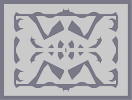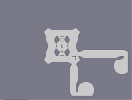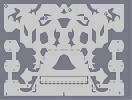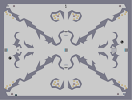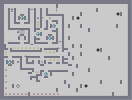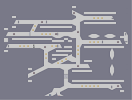Runic Survival Concept - eNergiser Defence Concept - Control Tower Utter Chaos Gunfight Tunnel Training

Pages: (0)

### finalmente, un revisar yo entiendo!

(hope i said that right, i didn't use a translator)

### Hey...

computer222's my favourite author! Quit hating.

### It doesn't matter how it's made

Nor does the genre of map matter either. However, I'm sorry to sound negative but I'm not finding this N-art even remotely interesting.

### I agree with you both guys, thanks

Do not give a shit like this map was made, I just like it.

fuck that guy

### featured maps are to demonstrate the cool things this game and its fans can do

absolutely every kind of map can and should be featured

### I don't see the problem with this being generated

A lot more effort went to the writing of the program than most n-arts / maps in general. To dismiss it because of that is short-sighted, IMO.

### haha Pony, it was practically as well,

http://www.literato.es/metaforas_de_desamor/
he should have made an auto-generated review!

### Agreed with Aidiera.

I'm not opposed to featured n-arts, but at least feature the ones with all that effort that typically goes into them.

### I don't think auto-generated

is a tag I want to see on a featured map.

is so hopeless

### and 'ahnelos' by 'anhelos'

:P

Certainly was not my day...

If you want to translate the review, you must replace 'probablememte' by 'probablemente' in the last sentence.

Nothing and nobody is perfect... :P

### Augh!!

You took my idea. Except this map was made a long time ago.

### Yeah, but.

He didn't write the program, I don't think.

### gahhhh

writing a program is art in itself! dont rate down for it. What matters is what the final product is! 5/5 this is awesome!

### Yeah, it's great

even though it was made by a tool. 4.5

### Yeah

Some colour would be good, but the actual image is in B&W, so it just came up with mines or gold.

### Looks cool

4/5

-1 for the tool.

Kind of inspires me to do something like this by hand. With some more color though.